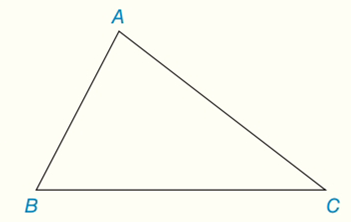Chapter 7.CT, Problem 6CT### Elementary Geometry for College St...

6th Edition
Daniel C. Alexander + 1 other
ISBN: 9781285195698

#### Solutions

Chapter
Section### Elementary Geometry for College St...

6th Edition
Daniel C. Alexander + 1 other
ISBN: 9781285195698
Textbook Problem
1 views

# For a given triangle (such as △ A B C ), what word describes the point of concurrency fora) the three angle bisectors? _________________b) the three medians? ___________________Exercises 6, 7

To determine

(a)

To describe:

The point of concurrency for the three angle bisectors for a given triangle (such as ABC).

Explanation

The incenter is the center of the triangle's incircle, the largest circle that will fit inside the triangle and touch all three sides.

One of several centers the triangle can have, the incenter is the point where the angle bisectors intersect. The incenter is also the center of the triangle's incircle- the largest circle that will fit inside the triangle.

Calculation:

The point where the three internal angle bisectors of a triangle are concurrent is called the incenter of the triangle.

Suppose we bisect the internal angles of ABC

To determine

(b)

To describe:

The point of concurrency for the three medians for a given triangle (such as ABC).

### Still sussing out bartleby?

Check out a sample textbook solution.

See a sample solution

#### The Solution to Your Study Problems

Bartleby provides explanations to thousands of textbook problems written by our experts, many with advanced degrees!

Get Started

#### In Exercises 1124, find the indicated limits, if they exist. 17. limx32x35

Applied Calculus for the Managerial, Life, and Social Sciences: A Brief Approach

#### Simplify: 1216

Elementary Technical Mathematics

#### Evaluate the expression sin Exercises 116. 3(2)0

Finite Mathematics and Applied Calculus (MindTap Course List)

#### Derive Equations 1 for the case /2 .

Single Variable Calculus: Early Transcendentals